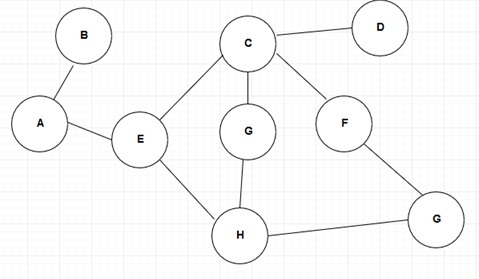# What is the Difference Between BFS and DFS

The main difference between BFS and DFS is that BFS or Breadth First Search proceeds level after level while DFS or Depth First Search follows a path from the starting to the end node and then moves to the other path from start to end and so on, until visiting all the nodes.

A graph is a nonlinear data structure that arranges data elements as a network model. Nodes in a graph are called vertices, and the edges connect these nodes. It is possible to solve most graph problems using search methods. Breadth First Search (BFS) and Depth First Search (DFS) are common search methods in use. In brief, BFS uses a queue while DFS uses a stack.

### Key Areas Covered

1. What is BFS
– Definition, Functionality
2. What is DFS
– Definition, Functionality
3. What is the Difference Between BFS and DFS
– Comparison of Key Differences

### Key Terms

BFS, DFS## What is BFS

BFS stands for Breath First Search. It uses a queue. The process is as follows.

• Visit the start vertex and place that element in the queue.
• Repeatedly remove a vertex from the queue visiting its unvisited adjacent vertices.
• Put newly visited vertices to the queue.

An example is as follows.Figure 1: BFS

Assume that the starting vertex in the above image is 1. The nodes connected to 1 are 2 and 4. So we can place 1, 2 and 4 in a queue. The output is 1, 2, 4. For 1, there are no remaining vertices. Therefore, we can remove 1 from the queue. Now we can move to 2. The adjacent vertices of 2 are 3, 5 and 6. Thus, we can place, 3, 5 and 6 in the queue. The output is 1, 2, 4, 3, 6.  In addition to these three numbers, there are no adjacent vertices connected to 2. So, we can remove 2 from the queue. Now, let us move to 4. The only adjacent node to 4 is 6. It has been visited already. There are no more vertices for 4. Therefore, we can remove 4 from the queue. You have to repeat this process until the queue is empty. It indicates the termination of the search operation.

## What is DFS

DFS stands for Depth First Search. The process is as follows.

Visit adjacent unvisited vertex and mark it as visited. Then, display it in output and push it into the stack.

If no adjacent vertex is found, pop up vertex from the stack.

Continue with above two steps until the stack is empty.Figure 2: DFS

The starting vertex is A. It is pushed into the stack. The adjacent vertices are B and E. Consider B. We can push B to the stack. As there are no adjacent nodes to B, we can pop B out of the stack. The output is A B.  The remaining adjacent node to A is E, so, we can pop E to the stack. Now the output is A B E.

As there are no adjacent nodes to A, we can pop ‘A’ out of the stack. The adjacent nodes for E are C and H. Now, consider C. We can push C to the stack. The output is A B E C. The process continues until the stack is empty. It terminates the search operation.

## Difference Between BFS and DFS

### Definition

BFS (Breadth first search) is a graph traversal algorithm that starts traversing the graph from the root node and explores all the neighboring nodes. DFS (Depth first search) is an algorithm that starts with the initial node of the graph and then goes deeper and deeper until finding the required node or the node which has no children. Thus, this is the main difference between BFS and DFS.

### Long Form

While BFS stands for Breadth First Search, DFS stands for Depth First Search.

### Method of Storing Nodes

Another major difference between BFS and DFS is that BFS uses queue while DFS uses stack.

### Memory Consumption

Moreover, BFS consumes more memory than DFS.

### Method of Traversing

Method of tranversing is another difference between BFS and DFS. BFS focuses on visiting the oldest unvisited vertices first while DFS focuses on visiting the vertices along the edge in the beginning.

### Conclusion

BFS and DFS are two search methods to find an element in a graph. The main difference between BFS and DFS is that BFS proceeds level after level while DFS follows a path from the starting to the end node and then moves to the other path from start to end and so on until visiting all the nodes.

##### Reference:

1.  Breadth First Search Algorithm | Pseudo Code | Introduction, Education 4u, 22 Mar. 2018, Available here.
2. Breadth First Search | BFS Examples |, Education 4u, 22 Mar. 2018, Available here.
3.  Depth First Search Algorithm | Graph Traversal Algorithm |, Education 4u, 22 Mar. 2018, Available here.
4. “BFS Algorithm – Javatpoint.” Www.javatpoint.com, Available here.
5. “DFS Algorithm – Javatpoint.” Www.javatpoint.com, Available here.